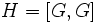# Characteristic not implies quasiautomorphism-invariant

This article gives the statement and possibly, proof, of a non-implication relation between two subgroup properties. That is, it states that every subgroup satisfying the first subgroup property (i.e., characteristic subgroup) need not satisfy the second subgroup property (i.e., quasiautomorphism-invariant subgroup)
View a complete list of subgroup property non-implications | View a complete list of subgroup property implications
EXPLORE EXAMPLES YOURSELF: View examples of subgroups satisfying property characteristic subgroup but not quasiautomorphism-invariant subgroup|View examples of subgroups satisfying property characteristic subgroup and quasiautomorphism-invariant subgroup

## Statement

It is possible to have a group$G$ and a characteristic subgroup$H$ of$G$ such that$H$ is not a quasiautomorphism-invariant subgroup, i.e., there are quasiautomorphisms of$G$ that do not send$H$ to itself.

## Proof

Further information: inner automorphism group of wreath product of groups of order p

Suppose$p$ is a prime number greater than$3$. Let$G$ be the group isomorphic to the inner automorphism group of the wreath product of two groups of order$p$.$G$ is a group of order$p^p$ with an elementary abelian normal subgroup$N$ of order$p^{p-1}$, an element of order$p$ acting on it from outside, and every non-identity element of$G$ has order$p$.

Consider the subgroup$H = [G,G]$.$H$ is a group of order$p^{p-2}$, contained inside the elementary abelian normal subgroup. It is a characteristic subgroup.

However, we can construct a quasiautomorphism$\sigma$ of$G$ that does not preserve$H$ as follows: the restriction of$\sigma$ to$N$ is an automorphism of$N$ that fixes the center$Z(G)$ (which is cyclic of order$p$) but does not send$H$ to itself, and$\sigma$ fixes every element of$G$ outside$H$. Note that we need$p > 3$ to ensure that$H$ is strictly bigger than$Z(G)$, which is necessary to be able to construct a$\sigma$ with the desired specifications.